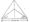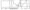# CSVTU Exams Questions Papers – Ist Year –Engineering Graphics– April-May-2009

BE (1st Year)

Examination, April-May-  2009

Engineering Graphics

1. (a) Explain Hypocycloid curve.

(b)   On a map, the distance between two points is 14 cm. The rec distance between them is 20 km. Draw a- diagonal scale c this map to read kilometers and hectameters and to measur upto 30 km. Show a distance of 18.9 km on this scale.

(c) Two fixed points A and B are 100 mm apart. Trace the complet path of a point P moving (in the same plane as that of A and E in such a way that, the sum of its distances from A and B i always the same and equal to 125 mm. Name the curve.

(d) A circle of 50 mm diameter rolls on the circumference of another circle of 175 mm diameter and outside it. Trace the locus of i point on the circumference of the rolling circle for one comple revolution. Name the curve.

Q.2.(a) Explain orthographic projection.

(b) A point 30 mm above xy line is the plan-view of two poi its P an Q. The elevation of P is 45 mm above the H.P. while that of Q i 35 mm below H.P. Draw the projections of the points and sta their position with reference to the principal plance and th quadrant in which they lie.

(c) In complete projections of a line PQ, inclined at 30° to thfe H.P. an given in the figure. Complete the projections and determine true length and its inclintion with the V.P.

Fig:-(d) Two oranges on a tree are, respectively 1.8m and 3m above the ground and 1.2m and 2.1m from 0.3m thick wall, tut on opposite sides of it. The distance between the oranges measUn along the ground and parallel to the wall is 2.7 m. Determine real distance between the oranges.

Q.3.(a) Explain Profile Plane.

(b) Draw the projections of a regular hexagon of 25 rn,n side, of its side in the H.R. and inclined at 60° to the VP anc making an angle of 45° with the HP.

(c) Draw the projections of a rhombus having diagonals 125 mm long the smaller diagonal of which is parallel principal planes, while the other is inclined at 30° to the HP.

(d) A triangular prism, base 30 mm side and axis 50mm lone the HP on one of its rectangular faces with its axis inclir the VP. It is cut by horizontal section plane at a distant above ground. Draw its front view and sectional top view.

Q.4.(a) Explain development of surfaces.

(b) Draw the development of the lateral surface of the p art P cone. The front view of the which is shown in fig.(d) Draw the isometric view from given orthographic predections:-

Fig:-

(d) Draw the isometric view from given orthographic projections.

Fig:-(b) Write any five basic commands of Auto CAD. Indicate the various options which are available in each command.

(c) Explain the functions of:

(i) Break                        (ii) Cal

(iii) Chamfer                 (iv) Change

(d) What are the benefits and limitations of CAD ?

### 1 thought on “CSVTU Exams Questions Papers – Ist Year –Engineering Graphics– April-May-2009”

1.10 years F.m.E. ques papers 1 st year